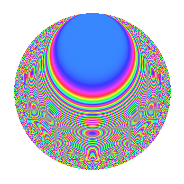# Properties

 Label 630.2.rLevel 630 Weight 2 Character orbit r Rep. character $$\chi_{630}(59,\cdot)$$ Character field $$\Q(\zeta_{6})$$ Dimension 96 Newform subspaces 2 Sturm bound 288 Trace bound 2

# Related objects

## Defining parameters

 Level: $$N$$ = $$630 = 2 \cdot 3^{2} \cdot 5 \cdot 7$$ Weight: $$k$$ = $$2$$ Character orbit: $$[\chi]$$ = 630.r (of order $$6$$ and degree $$2$$) Character conductor: $$\operatorname{cond}(\chi)$$ = $$315$$ Character field: $$\Q(\zeta_{6})$$ Newform subspaces: $$2$$ Sturm bound: $$288$$ Trace bound: $$2$$ Distinguishing $$T_p$$: $$13$$

## Dimensions

The following table gives the dimensions of various subspaces of $$M_{2}(630, [\chi])$$.

Total New Old
Modular forms 304 96 208
Cusp forms 272 96 176
Eisenstein series 32 0 32

## Trace form

 $$96q - 48q^{4} + 6q^{9} + O(q^{10})$$ $$96q - 48q^{4} + 6q^{9} + 6q^{14} + 2q^{15} - 48q^{16} + 10q^{21} + 6q^{24} - 6q^{29} + 2q^{30} + 30q^{35} + 6q^{41} - 12q^{44} + 18q^{45} + 6q^{46} - 12q^{49} - 36q^{50} - 16q^{51} - 10q^{60} - 18q^{61} + 96q^{64} - 54q^{65} - 48q^{66} + 84q^{69} + 6q^{70} - 30q^{75} - 74q^{81} - 26q^{84} + 66q^{89} + 54q^{90} + 66q^{95} - 6q^{96} - 52q^{99} + O(q^{100})$$

## Decomposition of $$S_{2}^{\mathrm{new}}(630, [\chi])$$ into newform subspaces

Label Dim. $$A$$ Field CM Traces $q$-expansion
$$a_2$$ $$a_3$$ $$a_5$$ $$a_7$$
630.2.r.a $$48$$ $$5.031$$ None $$-24$$ $$3$$ $$0$$ $$0$$
630.2.r.b $$48$$ $$5.031$$ None $$24$$ $$-3$$ $$0$$ $$0$$

## Decomposition of $$S_{2}^{\mathrm{old}}(630, [\chi])$$ into lower level spaces

$$S_{2}^{\mathrm{old}}(630, [\chi]) \cong$$ $$S_{2}^{\mathrm{new}}(315, [\chi])$$$$^{\oplus 2}$$

## Hecke Characteristic Polynomials

There are no characteristic polynomials of Hecke operators in the database